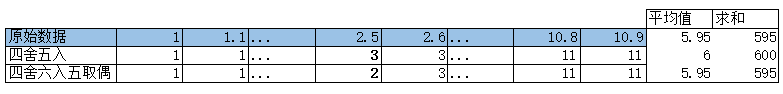.Net开发遇到的趣事。

# — 问题 —

Math.Round(3.5);返回的结果是4
Math.Round(4.5);返回的结果是4

# — 解决 —

Math.Round(4.5, MidpointRoundind.ToEven); //四舍六入、五取偶, 返回结果是4
Math.Round(4.5, MidpointRoundind.AwayFromZero); //四舍六入、五远离零（即四舍五入），返回结果是5

# — 深挖 —

IEEE 754: https://en.wikipedia.org/wiki/IEEE_754#Rounding_rules

IEEE 754标准的写明了：

### Roundings to nearest

Round to nearest, ties to even – rounds to the nearest value; if the number falls midway it is rounded to the nearest value with an even (zero) least significant bit; this is the default for binary floating-point and the recommended default for decimal.

Round to nearest, ties away from zero – rounds to the nearest value; if the number falls midway it is rounded to the nearest value above (for positive numbers) or below (for negative numbers); this is intended as an option for decimal floating point.### 1 条回复

1.antior说道：

很厉害的样子啊。